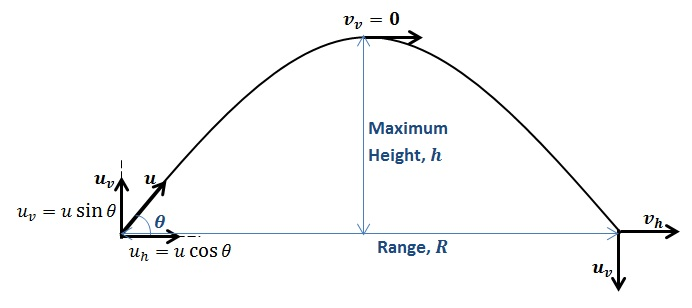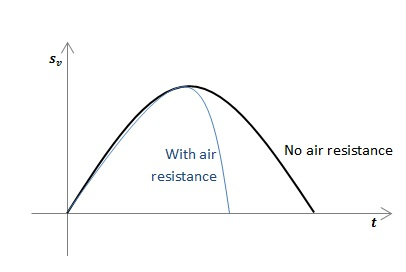# How to Solve Projectile Motion Problems

Projectiles are motions involving two dimensions. To solve projectile motion problems, take two directions perpendicular to each other (typically, we use the “horizontal” and the “vertical” directions) and write all vector quantities (displacements, velocities, accelerations) as components along each of these directions. In projectiles, the vertical motion is independent of the horizontal motion. So, equations of motion can be applied to horizontal and vertical motions separately.

To solve projectile motion problems for situations where objects are thrown on Earth, the acceleration due to gravity,$g=9.81\mathrm{\:m\:s^{-1}}$, is always acting vertically downward. If we neglect effects of air resistance, then the horizontal acceleration is 0. In this case, the horizontal component of the projectile’s velocity remains unchanged.

When a projectile thrown at an angle reaches the maximum height, its vertical component of velocity is 0 and when the projectile reaches the same level from which it was thrown, its vertical displacement is 0On the diagram above, I have shown some typical quantities you should know in order to solve projectile motion problems.$u$ is the initial velocity and$v$, is the final velocity. The subscripts$h$ and$v$ refer to the horizontal and vertical components of these velocities, separately.

In doing the following calculations, we take upwards direction to be positive in the vertical direction, and horizontally, we take vectors to the right to be positive.

Let us consider the vertical displacement of the particle with time. The initial vertical velocity is$u_v$. At a given time, the vertical displacement$s_v$, is given by$s_v=u_vt-\frac{1}{2}gt^2$. If we are to draw a graph of$s_v$ vs.$t$, we find that the graph is a parabola because$s_v$ has a dependence on$t^2$. i.e., the path taken by the object is a parabolic one.

Strictly speaking, due to air resistance, the path is not parabolic. Rather, the shape becomes more “squashed”, with the particle getting a smaller range.Initially, the object’s vertical speed is decreasing since the Earth is trying to attract it downwards. Eventually, the vertical speed reaches 0. The object has now reached the maximum height. Then, the object starts to move downwards, its downwards velocity increasing as the object is accelerated downwards by gravity.

For an object thrown from the ground at speed$u$, let’s try to find the time taken for the object to reach the top. To do this, let’s consider the motion of the ball from when it was thrown to when it reaches the maximum height.

The vertical component of the initial velocity is$u_v$. When the object reaches the top, the object’s vertical velocity is 0. i.e.$v_v=0$. According to the equation$v_v=u_v-gt$, the time taken to reach the top =$t=\frac{u_{v}}{g}$.

If there is no air resistance, then we have a symmetrical situation, where the time taken for the object to reach the ground from its maximum height is equal to the time taken by the object to reach the maximum height from the ground in the first place. The total time that the object spends in air is then,$t=\frac{2u_{v}}{g}$.

If we consider the object’s horizontal motion, we can find the object’s range. This is the total distance traveled by the object before it lands on the ground. Horizontally,$s=ut+\frac{1}{2}at^2$ becomes$R=u_ht$ (because horizontal acceleration is 0). Substituting for$t$, we have:$t=\frac{2u_{h}u_{v}}{g}$.

Example 1

A person standing at the top of a building 30 m tall throws a rock horizontally from the edge of the building at the speed of 15 m s-1. Find

a) the time taken by the object to reach the ground,

b) how far away from the building it lands, and

c) the speed of the object when it reaches the ground.

The horizontal velocity of the object does not change, so this isn’t useful by itself to calculate the time. We know the vertical displacement of the object from the top of the building to the ground. If we can find the time taken by the object to reach the ground, we can then find how much the object should move horizontally during that time.

So, let us start with the vertical motion from when it was thrown to when it reaches the ground. The object is thrown horizontally, so the initial vertical velocity of the object is 0. The object would experience a constant vertical acceleration downwards, so$a_v=g=-9.81$ m s-2. The vertical displacement for the object is$s_v=-30$ m. Now we use$s=ut+\frac{1}{2}at^2$, with$-30=-\frac{1}{2}\times9.81\times t^{2}$. So,$t=\sqrt{\frac{2\times30}{9.81}}=\mathrm{6.12\: s}$.

To solve part b) we use horizontal motion. Here, we have$u_h=$ 15 m s-1,$t=$ 6.12 s, and$a_h=$ 0. Because horizontal acceleration is 0, the equation$s_h=u_ht+\frac{1}{2}a_ht^2$ becomes$s_h=u_ht$ or,$s_h=15\times6.12=91.8\mathrm{\:m}$. This is how much farther from the building the object would land.

To solve part c) we need to know the final vertical and horizontal velocities. We already know the final horizontal velocity,$v_h=15$ m s-1. We need to again consider the vertical motion to know the object’s final vertical velocity,$v_v$. We know that$u_v=0$,$s_v=$ -30 m and$a_v=g=-9.81$ m s-2. Now we use$v^2=u^2+2as$, giving us${\left(v_v\right)}^2=0^2-2\times9.81\times(-15)$. Then,$v_v=\sqrt{2\times9.81\times15}=17.2\mathrm{\: m\: s^{-1}}$. Now we have the horizontal and vertical components of the final speed. The final speed is, then,$v=\sqrt{{v_h}^2+{v_v}^2}= \sqrt{15^{2}+17.2^{2}}=22.8$ m s-1.

Example 2

A football is kicked off the ground at a speed f 25 m s-1, with an angle of 20o to the ground. Assuming there is no air resistance, find how much farther away the ball will land.

This time, we have a vertical component for initial velocity too. This is,$u_v=25\mathrm{sin}20^{o}=8.55$ m s-1. The initial horizontal velocity is$u_h=25\mathrm{cos}20^{o}=23.5$ m s-1.

When the ball lands, it comes back to the same vertical level. So we can use$s_v=u_vt+\frac{1}{2}a_vt^2$, with$s_v=0$. This gives us$0=8.55t+\frac{1}{2}\times(-9.81)t^{2}$. Solving the quadratic equation, we get a time of$t=$ 0 s or 1.74 s. Since we are looking for the time when the ball lands, we take$t=$ 1.74 s.

Horizontally, there is no acceleration. So we can substitute the time of the ball’s landing into the horizontal equation of motion:$s_{h}=u_{h}t=23.5\times1.74=40.9$ m. This is how far away the ball will land.